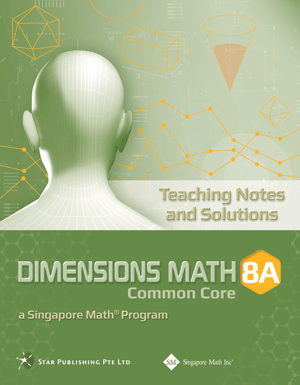# Singapore Math Dimensions Math Teaching Notes and Textbook Solutions 8A

\$ 31.00

Dimensions Math® Textbook 8A

Chapter 1: Exponents and Scientific Notation

1.1 Positive Exponents and the Laws of Exponents
1.2 Zero and Negative Integer Exponents
1.3 Fractional Exponents

A. nth root of a Positive Number
B. Fractional Exponents

1.4 Comparing Exponents
1.5 Scientific Notation

A. Representation of Very Small or Very Large Numbers
B. Computation

1.6 Rounding Numbers to a Specified Number of Significant Figures
1.7 Estimations and Accuracy of Calculators

A. Estimations
B. Estimation and Irrational Numbers
C. Accuracy in Calculators

In a Nutshell
Review Exercise 1

Chapter 2: Linear Equations in Two Variables

2.1 Linear Equations in Two Variables

A. Review of Linear Equations in One Variable
B. Meaning of Linear Equations in Two Variables

2.2 Solving Simultaneous Linear Equations in Two Variables using the Graphical Method
2.3 Solving Simultaneous Linear Equations in Two Variables using the Substitution Method
2.4 Solving Simultaneous Linear Equations in Two Variables using the Elimination Method
2.5 Solving Problems using Simultaneous Equations

In A Nutshell
Review Exercise 2

Chapter 3: Expansion and Factorization of Algebraic Expressions

3.1 Expansion of the Products of Algebraic Expressions

A. Product of Single Terms
B. Product of Two Expressions

3.2 Special Products More Properties of Inequalities
3.3 Factorization by using Special Products of Algebraic Expressions

In A Nutshell
Review Exercise 3

Chapter 4: Quadratic Factorization and Equations

4.1 Factorization of ax2 + bx +c

A. x2 + bx + c
B. ax2 + bx + c

4.2 Solving Quadratic Equations by Factorization
4.3 Problem Solving Involving Quadratic Equations

In A Nutshell
Review Exercise 4

Chapter 5: Simple Algebraic Fractions

5.1 Simplifying Simple Algebraic Fractions
5.2 Multiplication and Division of Algebraic Fractions
5.3 Addition and Subtraction of Algebraic Fractions
5.4 Fractional Equations

A. Solving Equations Involving Algebraic Fractions
B. Problem Solving Involving Fractional Equations

A. Finding the Value of an Unknown Quantity in a Formula
B. Changing the Subject of a Formula

In A Nutshell
Review Exercise 5

Chapter 6: Congruence and Reflections

6.1 Congruence and Reflections

A. Congruent Figures
B. Reflections

6.2 Translations, Rotations and Combining Transformations

A. Translations
B. Rotations
C. Combining Transformations

6.3 Similarity
6.4 Enlargements and Similarity

A. Scale Factors
B. Enlargements

In A Nutshell
Review Exercise 6

Chapter 7: Parallel Lines and Angles in Triangles and Polygons

7.1 Angles formed by Parallel Lines and Transversals

A. Angle Properties
B. Parallel Lines and Transversals

7.2 Interior and Exterior Angles of a Triangle

A. Angle Sum of a Triangle
B. Exterior Angle of a Triangle

7.3 Angles Properties of Special Quadrilaterals
7.4 Polygons

A. Types of Polygons
B. Sum of Interior Angels of a Polygons
C. Sum of Exterior Angels of a Polygons

In A Nutshell
Review Exercise 7

Click here to view Dimensions Math® Teaching Notes and Solutions 8A samples.

Dimensions Math® G6-8 Textbooks are designed for middle school students. Developed in collaboration between Star Publishing and Singapore Math Inc®, this series follows the Singapore Mathematics Framework and covers the topics in the Common Core State Standards.

The emphasis of this series is on empowering the students to learn mathematics effectively and independently. Depending on the topics covered, different approaches are adopted for the presentation of concepts to facilitate easy understanding by students to internalize concepts and instill in them an interest to explore the topics further.

Each book includes appropriate examples, class activities and diagrams to understand the concepts and apply them. IT skills and values are incorporated as appropriate.

Some features of this series include the following:

1. Class activities which allow the student to learn mathematics through discovery. Some of the class activities make use of Geometer’s Sketchpad (available at keypress.com).
2. Worked examples are followed by a similar question (Try It!) so that students can check if they have understood the concepts presented earlier.
3. An exercise for each lesson including questions in the following sequence:
• Basic Practice (simple questions involving a direct application of the concepts)
• Further Practice (more challenging questions on direct application)
• Maths@Work (questions that apply mathematical concepts to real-life situations)
• Brainworks (questions involving higher order thinking or an open-ended approach to problems)
4. Each chapter is followed by a review exercise; the Extend Your Learning Curve activity encourages students to explore mathematical concepts further or apply mathematics in real-life situations, with some questions requiring sentence or paragraph answers to encourage students to reflect on their learning experiences.
5. The right margins contain remarks on important information, concepts or definitions covered earlier related to the current material to help students recall them, discussion questions, interesting puzzles or facts that are related to mathematics, and some website references.

The answer key at the back of the book provides answers to the Try It! and the problems in the exercises for the Basic PracticeFurther Practice, and Maths@Work questions. It does not include answers to the class activities or the Extend Your Learning Curve activities.